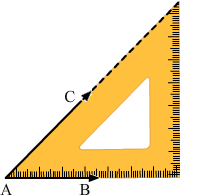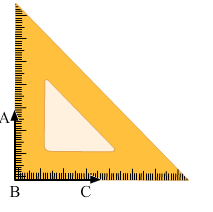# RD Sharma Class 6 ex 18.1 Solutions Chapter 18 Basic Geometrical Tools

In this chapter, we provide RD Sharma Class 6 ex 18.1 Solutions Chapter 18 Basic Geometrical Tools for English medium students, Which will very helpful for every student in their exams. Students can download the latest RD Sharma Class 6 ex 18.1 Solutions Chapter 18 Basic Geometrical Tools Maths pdf, Now you will get step by step solution to each question.

# Chapter 18: Basic Geometrical Tools Exercise 18.1

### Question: 1

Construct the following angles using set- squares:

(i) 45°

(ii) 90°

(iii) 60°

(iv) 105°

(v) 75°

(vi) 150°

### Solution:(i) 45°

Place 45° set- square.

Draw two rays AB and AC along the edges from the vertex from the vertex of 45angle of the set- square.

The angle so formed is a 45° angle.

∠BAC = 45°

(ii) 90°

Place = 90° set –square as shown in the figure.Draw two rays BC and BA along the edges from the vertex of 90° angle.

The angle so formed is 90° angle.

∠ABC = 90°

(iii) 60°

Place 30° set –square as shown in the figure.

Draw the rays BA and BC along the edges from the vertex of 60°

The angle so formed is 60°

∠ABC = 60°

(iv) 105°

Place 30° set –square and make an angle 60° by drawing the rays BA and BC as shown in figure.

Now place the vertex of 45°of the set –square on the ray BA as shown in figure and draw the ray BD.

The angle so formed is 105°

Therefore, ∠DBC = 105°

(v) 75o

Place 45° set –square and make an angle of 45° by drawing the rays BD and BC as shown in the figure.

Now place the vertex of 30° of the set- square on the ray BD as shown in the figure and draw the ray BA.

The angle so formed is 75°.

Therefore, ∠ABC = 75°

(Line BD is hidden)

(vi) 150°

Place the vertex of 45° of the set – square and make angle of 90o by drawing the rays BD and BC as shown in the figure

Now, place the vertex of 30°of the set –square on the ray BS as shown in the figure and draw the ray BA

The angle so formed is 150°.

Therefore, ∠ABC = 150°

### Question: 2

Given a line BC and a point A on it, construct a ray AD using set – squares so that ∠DAC is

(i) 30°

(ii) 150°

### Solution:

(i) Draw a line BC and take a point A on it. Place 30° set –square on the line BC such that its vertex of 30° angle lies on point A and one edge coincides with the ray AB as shown in figure

Thus ∠DAC is the required angle of 30°

(ii) Draw a line BC and take a point A on it. Place 30° set –square on the line BC such that its vertex of 30° angle lies on point A and one edge coincides with the ray AB as shown in the figure.